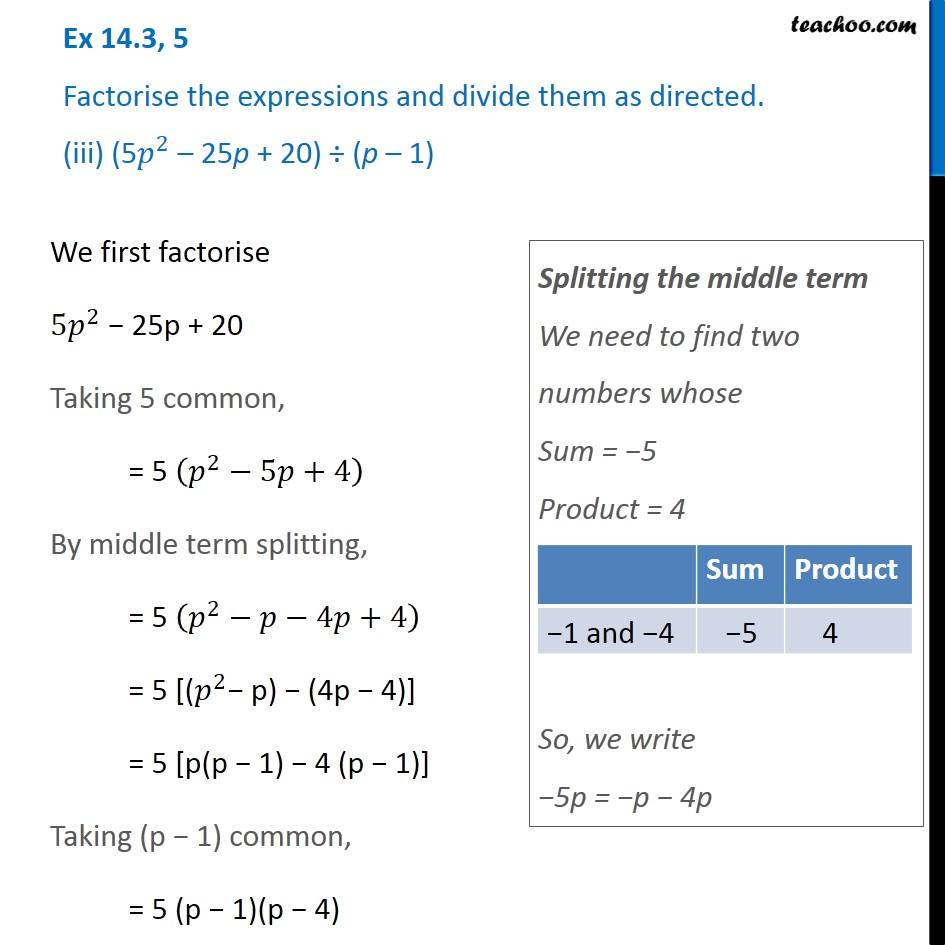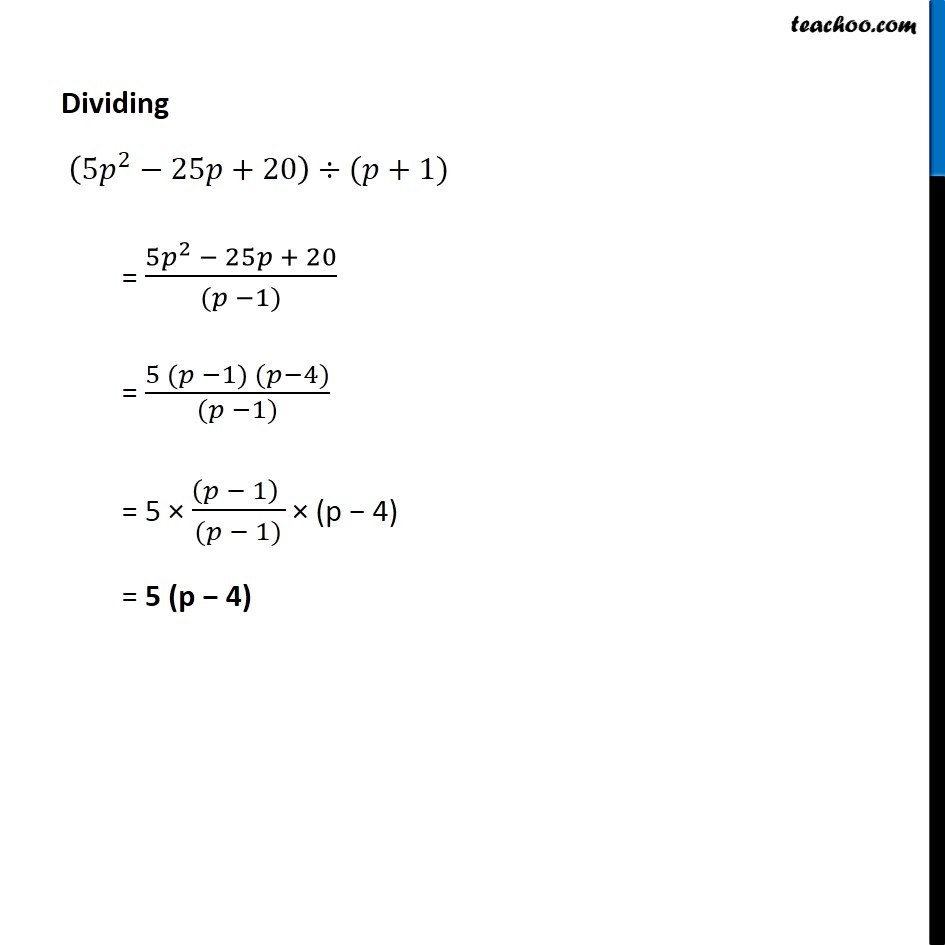Dividing two polynomials

Chapter 14 Class 8 Factorisation
Concept wiseIntroducing your new favourite teacher - Teachoo Black, at only ₹83 per month

### Transcript

Ex 14.3, 5 Factorise the expressions and divide them as directed. (iii) (5𝑝^2 – 25p + 20) ÷ (p – 1) We first factorise 〖5𝑝〗^2 − 25p + 20 Taking 5 common, = 5 (𝑝^2−5𝑝+4) By middle term splitting, = 5 (𝑝^2−𝑝−4𝑝+4) = 5 [(𝑝^2− p) − (4p − 4)] = 5 [p(p − 1) − 4 (p − 1)] Taking (p − 1) common, = 5 (p − 1)(p − 4) Splitting the middle term We need to find two numbers whose Sum = −5 Product = 4 So, we write −5p = −p − 4p Dividing (〖5𝑝〗^2−25𝑝+20)÷(𝑝+1) = (〖5𝑝〗^2 − 25𝑝 + 20)/((𝑝 −1)) = (5 (𝑝 −1) (𝑝−4))/((𝑝 −1)) = 5 × ((𝑝 − 1) )/((𝑝 − 1)) × (p − 4) = 5 (p − 4)